# Attribute-value system facts for kids

Kids Encyclopedia Facts

An attribute-value system is a basic knowledge representation framework comprising a table with columns designating "attributes" (also known as "properties", "predicates," "features," "dimensions," "characteristics" or "independent variables" depending on the context) and rows designating "objects" (also known as "entities," "instances," "exemplars," "elements" or "dependent variables."). Each table cell therefore designates the value (also known as "state") of a particular attribute of a particular object.

## Example of attribute-value system

Below is a sample attribute-value system. It represents 10 objects (rows) and five features (columns). In this example, the table contains only integer values. In general, an attribute-value system may contain any kind of data, numeric or otherwise. An attribute-value system is distinguished from a simple "feature list" representation in that each feature in an attribute-value system may possess a range of values (e.g., feature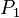$P_{1}$ below, which has domain of {0,1,2}), rather than simply being present or absent (Barsalou & Hale 1993)

Sample Attribute-Value System
Object$P_{1}$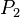$P_{2}$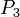$P_{3}$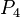$P_{4}$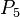$P_{5}$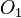$O_{1}$ 1 2 0 1 1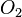$O_{2}$ 1 2 0 1 1$O_{3}$ 2 0 0 1 0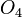$O_{4}$ 0 0 1 2 1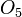$O_{5}$ 2 1 0 2 1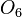$O_{6}$ 0 0 1 2 2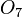$O_{7}$ 2 0 0 1 0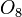$O_{8}$ 0 1 2 2 1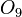$O_{9}$ 2 1 0 2 2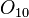$O_{10}$ 2 0 0 1 0

## Other terms used for "attribute-value system"

Attribute-value systems are pervasive throughout many different literatures, and have been discussed under many different names:

• Flat data
• Attribute-value system (Ziarko & Shan 1996)
• Information system (Pawlak 1981)
• Classification system (Ziarko 1998)
• Knowledge representation system (Wong & Ziarko 1986)
• Information table (Yao & Yao 2002)
• Object-predicate table (Watanabe 1985)
• Aristotelian table (Watanabe 1985)
• Simple frames (Barsalou & Hale 1993)
• First normal form database

## Related pages

• Bayes networks
• Entity-Attribute-Value model
• Joint distribution
• Knowledge representation
• Optimal classification
• Rough set
• Barsalou, Lawrence W. & Christopher R. Hale (1993), "Components of conceptual representation: From feature lists to recursive frames", in Iven Van Mechelen, James Hampton, Ryszard S. Michalski, & Peter Theuns, Categories and Concepts: Theoretical Views and Inductive Data Analysis, London: Academic PressAttribute-value system Facts for Kids. Kiddle Encyclopedia.